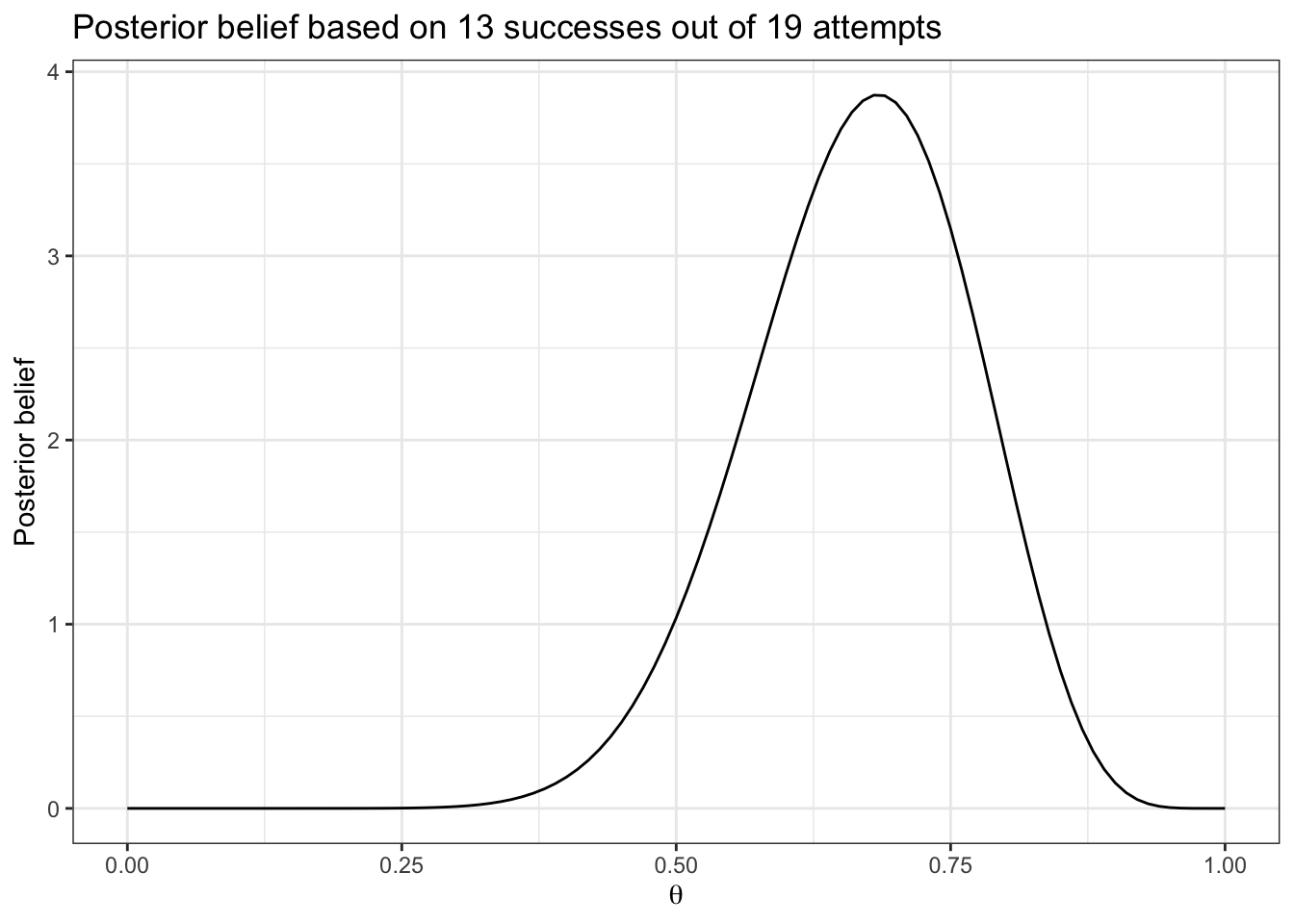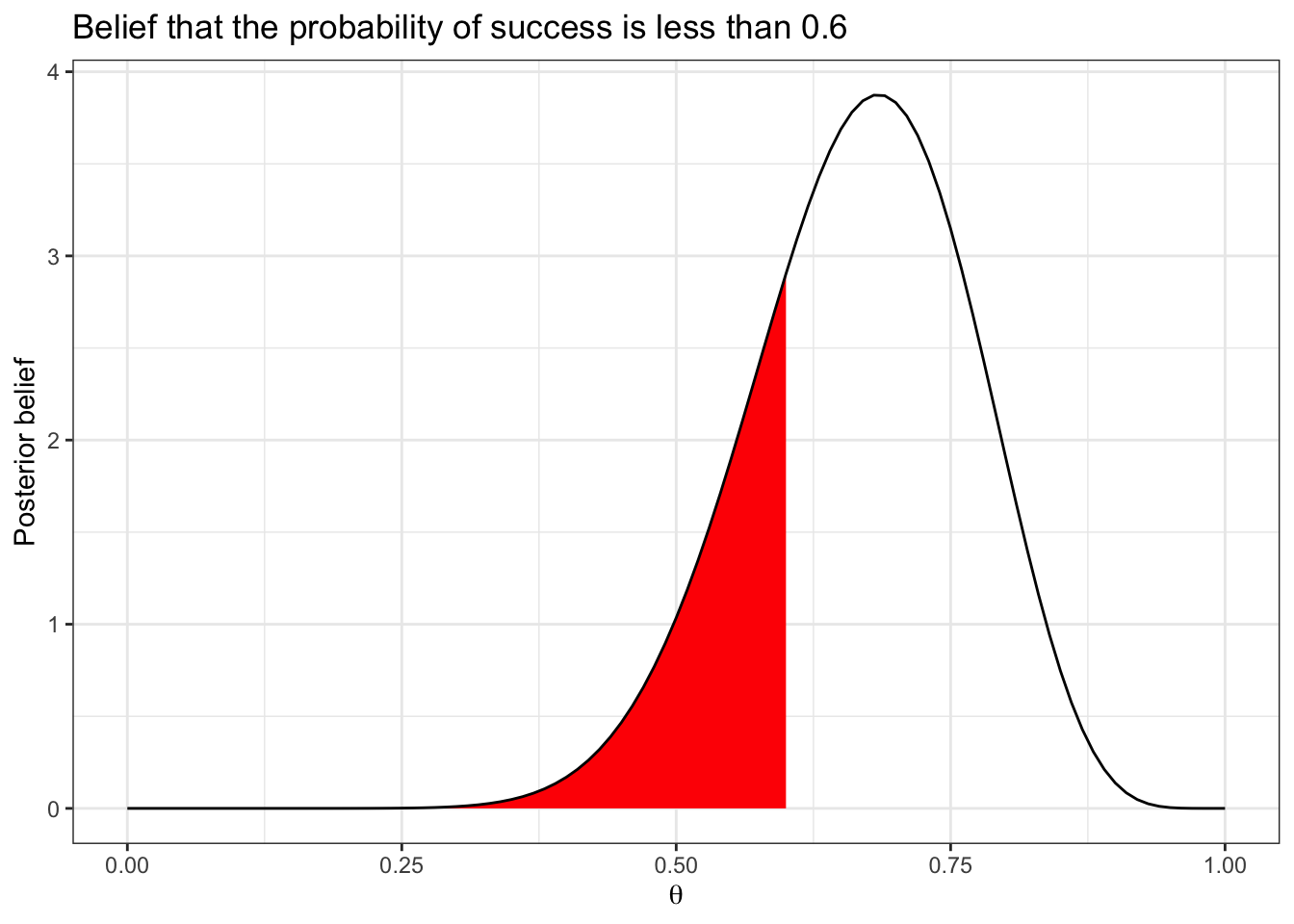This page provides a summary of statistical methods in R used to analyze binomial data. It begins by considering a single group and then expands to multiple groups. For completing course activities, you should copy relevant code from here and modifying it as necessary.

In the code below, we will make use of the tidyverse package, so make sure to load it at the beginning of your R session.

library("tidyverse")

## Single group

Binomial data, written $$Y \sim Bin(n,\theta)$$ is characterized by

• $$y$$ successes out of $$n$$ attempts,
• common probability of success $$\theta$$,
• and each attempt is independent.

If our sample is based on a random sample from our population, then we can make inferences about that entire population.

Suppose we observe 13 successes out of 19 attempts. In R, we would write

y <- 13
n <- 19

A point estimate divides the 13 successes by the 19 attempts, i.e.

y/n
##  0.6842105

Our posterior belief about the unknown probability of success ($$\theta$$) is represented by the following curve

d <- data.frame(x = seq(from = 0, to = 1, length = 1001))

ggplot(d) +
stat_function(fun = dbeta,
args = list(shape1 = 1+y, shape2 = 1+n-y)) +
labs(x = expression(theta),
y = "Posterior belief",
title = "Posterior belief based on 13 successes out of 19 attempts")We can calculate probabilities based on this curve by finding the area under the curve. For example, to find the probability that $$\theta$$ is less than 0.6 we use

pbeta(0.6, shape1 = 1+y, shape2 = 1+n-y)
##  0.2500107

which corresponds to the area

d <- data.frame(x = seq(from = 0, to = 1, length = 1001))

ggplot(d) +
stat_function(fun = dbeta, xlim = c(0,0.6), geom = "area", fill = "red",
args = list(shape1 = 1+y, shape2 = 1+n-y)) +
stat_function(fun = dbeta,
args = list(shape1 = 1+y, shape2 = 1+n-y)) +
labs(x = expression(theta),
y = "Posterior belief",
title = "Belief that the probability of success is less than 0.6")To calculate the probability of being above 0.6 use

1-pbeta(0.6, shape1 = 1+y, shape2 = 1+n-y)
##  0.7499893

A 95% credible interval is calculated using

a <- 0.05
qbeta(c(a/2, 1-a/2), shape1 = 1+y, shape2 = 1+n-y)
##  0.4572108 0.8460908

## Multiple groups

Binomial data for multiple groups is written $$Y_g \sim Bin(n_g,\theta_g)$$ for $$i=1,\ldots,G$$ where $$G$$ is the number of groups. For group $$g$$, we have

• $$y_g$$ successes out of $$n_g$$ attempts,
• common within-group probability of success $$\theta_g$$,
• and every attempt is independent.

If our sample is based on a random sample from our population, then we can make inferences about that entire population.

Suppose we observe 13 success out of 19 attempts in group 1 and 23 success out of 25 attempts in group 2. We may code this up as

d <- tribble(
~group, ~successes, ~attempts,
1,         13,        19,
2,         23,        25
)

We can determine point estimates using

d %>% mutate(point_estimate = successes/attempts)
## # A tibble: 2 × 4
##   group successes attempts point_estimate
##   <dbl>     <dbl>    <dbl>          <dbl>
## 1     1        13       19          0.684
## 2     2        23       25          0.92

We can plot our posterior beliefs using the following code

# Function to create the posterior
create_posterior <- function(d) {
y <- d$successes n <- d$attempts

data.frame(theta = seq(0, 1, length=1001)) %>%
mutate(posterior = dbeta(theta, shape1 = 1+y, shape2 = 1+n-y))
}

# Construct the curves
curves <- d %>%
group_by(group) %>%
do(create_posterior(.)) %>%
mutate(group = factor(group)) # so that we can use it as a linetype

# Plot curves
ggplot(curves, aes(x = theta, y = posterior, color = group, linetype = group)) +
geom_line() +
labs(x = expression(theta),
y = "Posterior belief",
title = "Posterior beliefs for two groups.") +
theme_bw()